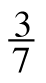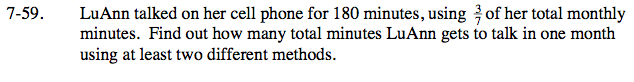Home > CC2 > Chapter 7 > Lesson 7.1.5 > Problem7-59

7-59.

LuAnn talked on her cell phone for 180 minutes, usingof her total monthly minutes. Find out how many total minutes LuAnn gets to talk in one month using at least two different methods. Homework Help ✎$180\text{ minutes is }\frac{3}{7}\text{ of her total}$

$\text{monthly minutes.}\;\;\;\;\;\;\;\;\;\;\;\;\;\;\;\;\;\;$

$180=\frac{3}{7}x$

$\left(\frac{7}{3}\right)180=\left(\frac{7}{3}\right)\frac{3}{7}x$

x = 420 minutes